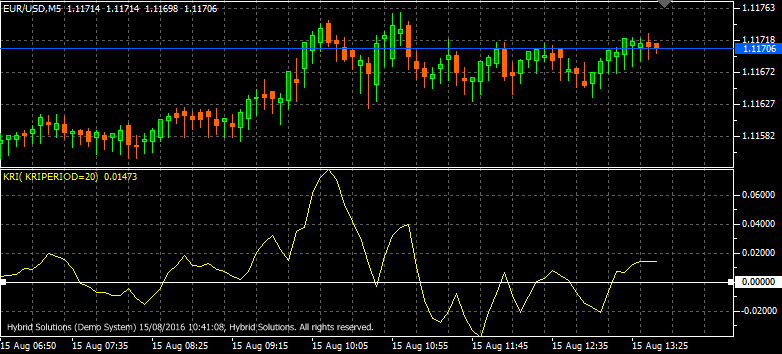# KRI

Free

Developed By: OmSaiTech

In stock

KRI Candle indicator is a simple yet powerful VertexFX client-side indicator that is based on Moving Averages.

SKU: 1248 Categories: ,KRI Candle indicator is a simple yet powerful VertexFX client-side indicator that is based on Moving Averages.

At first we calculate the Moving Average Envelope over the KRIPeriod of the High if MODE is 1, and the Low if the MODE is set to 2. In the second step, we calculate the KRI, which is ratio of difference between the current price and the Moving Average envelope, divided by the Moving Average envelope. This value is then expressed as percentage.

When the indicator value is above zero, it implies bullish trend, and traders can initiate Long positions. When the indicator has peaked out above zero and is gradually falling, traders should exit their Long positions. When the indicator value is below zero, it implies Bearish trend, and traders can initiate Short positions. When the indicator has bottomed out below zero and is gradually rising, traders should exit their Short positions.

Configurable Inputs
1. KRIPeriod – The number of bars used to calculate the KRI period.
2. MODE –The mode used to calculate the Moving Average Envelope. The allowed values are 1 (HIGH) and 2(LOW), defined by the INDICATOR_LINESenumeration.
3. MA_TYPE – The method used to calculate the Moving Average used within the KRI indicator as defined by the ENUM_MA_METHODenumeration. The allowed values are 1 (SMA – Simple Moving Average), 2 (EMA – Exponential Moving Average), 3 (TSMA – Time Series Moving Average), 4 (VMA – Variable Moving Average), 5 (TMA – Triangular Moving Average), 6 (VIDYA) and 7 (Mode Weighted).